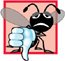# Confusing Equality (==) and Assignment (=) Operators

There is one type of error that C++ programmers, no matter how experienced, tend to make so frequently that we feel it requires a separate section. That error is accidentally swapping the operators == (equality) and = (assignment). What makes these swaps so damaging is the fact that they ordinarily do not cause syntax errors. Rather, statements with these errors tend to compile correctly and the programs run to completion, often generating incorrect results through runtime logic errors. [Note: Some compilers issue a warning when = is used in a context where == normally is expected.]

There are two aspects of C++ that contribute to these problems. One is that any expression that produces a value can be used in the decision portion of any control statement. If the value of the expression is zero, it is treated as false, and if the value is nonzero, it is treated as TRue. The second is that assignments produce a valuenamely, the value assigned to the variable on the left side of the assignment operator. For example, suppose we intend to write

```if ( payCode == 4 )
cout << "You get a bonus!" << endl;
```

but we accidentally write

```if ( payCode = 4 )
cout << "You get a bonus!" << endl;
```

The first if statement properly awards a bonus to the person whose payCode is equal to 4. The second if statementthe one with the errorevaluates the assignment expression in the if condition to the constant 4. Any nonzero value is interpreted as TRue, so the condition in this if statement is always true and the person always receives a bonus regardless of what the actual paycode is! Even worse, the paycode has been modified when it was only supposed to be examined!

Common Programming Error 5.14Using operator == for assignment and using operator = for equality are logic errors.

Error-Prevention Tip 5.3Programmers normally write conditions such as x == 7 with the variable name on the left and the constant on the right. By reversing these so that the constant is on the left and the variable name is on the right, as in 7 == x, the programmer who accidentally replaces the == operator with = will be protected by the compiler. The compiler treats this as a compilation error, because you can't change the value of a constant. This will prevent the potential devastation of a runtime logic error.

Variable names are said to be lvalues (for "left values") because they can be used on the left side of an assignment operator. Constants are said to be rvalues (for "right values") because they can be used on only the right side of an assignment operator. Note that lvalues can also be used as rvalues, but not vice versa.

There is another equally unpleasant situation. Suppose the programmer wants to assign a value to a variable with a simple statement like

```x = 1;
```

```x == 1;
```

Here, too, this is not a syntax error. Rather, the compiler simply evaluates the conditional expression. If x is equal to 1, the condition is true and the expression evaluates to the value true. If x is not equal to 1, the condition is false and the expression evaluates to the value false. Regardless of the expression's value, there is no assignment operator, so the value simply is lost. The value of x remains unaltered, probably causing an execution-time logic error. Unfortunately, we do not have a handy trick available to help you with this problem!

Error-Prevention Tip 5.4Use your text editor to search for all occurrences of = in your program and check that you have the correct assignment operator or logical operator in each place.C++ How to Program (5th Edition)
ISBN: 0131857576
EAN: 2147483647
Year: 2004
Pages: 627